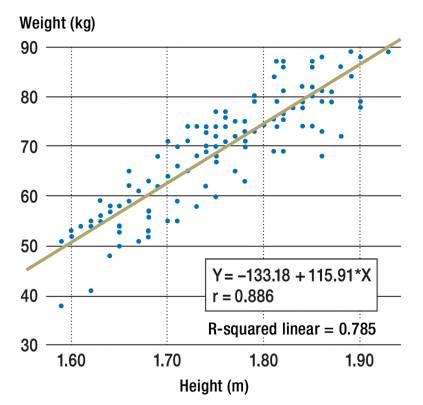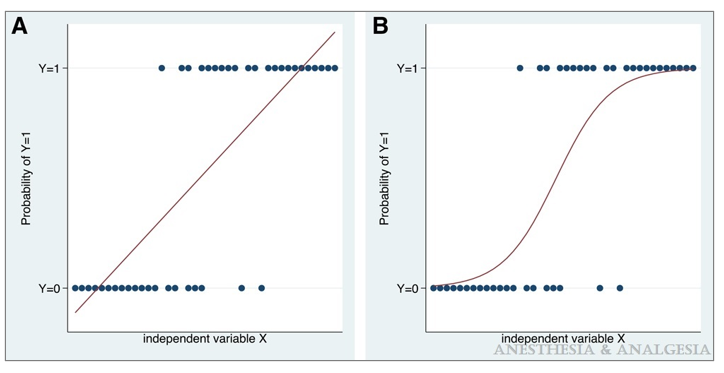# Machine Learning Algorithms

In this blog post, we are going to be discussing machine learning algorithms. We will be giving a brief overview of what machine learning is, and then talking about different types of algorithms that you can use in the field. Finally, we will provide some examples of how these algorithms can be used.

## What is machine learning

Machine learning is a field of computer science that allows computers to learn from data without being explicitly programmed. In other words, machine learning algorithms automatically improve given experience. For example, consider a child who learns to recognize birds. After seeing many examples of birds, the child develops a set of rules for distinguishing birds from other objects. This process of learning from experience is similar to what happens in machine learning. The main machine learning types are supervised learning, unsupervised learning and reinforcement learning. More on Types of Machine Learning post.

## Supervised Learning Algorithms

### Linear RegressionSource: Linear Regression Analysis -Schneider A, Hommel G, Blettner M. Linear regression analysis: part 14 of a series on evaluation of scientific publications. Dtsch Arztebl Int. 2010 Nov;107(44):776-82. doi: 10.3238/arztebl.2010.0776. Epub 2010 Nov 5. PMID: 21116397; PMCID: PMC2992018.A scatter plot and the corresponding regression line and regression equation for the relationship between the dependent variable body weight (kg) and the independent variable height (m).r = Pearsons’s correlation coefficientR-squared linear = coefficient of determination

The linear regression machine learning algorithm is a supervised learning algorithm that is used to predict a continuous target variable based on one or more predictor variables. The algorithm assumes that there is a linear relationship between the predictor variables and the target variable, and it attempts to find the line of best fit. The error term is also assumed to be normally distributed. The advantages of the linear regression algorithm include its simplicity and interpretability. Additionally, the algorithm can be applied to a wide range of problems. However, the algorithm is also limited by its assumption of linearity, and it may not be well suited for problems with nonlinear relationships.

### Logistic RegressionSource: Logistic Regression in Medical Research (Schober, Patrick MD, PhD, MMedStat*; Vetter, Thomas R. MD, MPH†)Relationship between a continuous independent variable X and a binary outcome that can take values 0 (eg, “no”) or 1 (eg, “yes”). As shown, the probability that the value of the outcome is 1 seems to increase with increasing values of X. A, Using a straight line to model the relationship of the independent variable with the probability provides a poor fit; results in estimated probabilities <0 and >1; and grossly violates the assumptions of linear regression. Logistic regression models a linear relationship of the independent variable with the natural logarithm (ln) of the odds of the outcome variable. B, This translates to a sigmoid relationship between the independent variable and the probability of the outcome being 1, with predicted probabilities appropriately constrained between 0 and 1.

Logistic regression is a machine learning algorithm used for classification problems. It is a linear model that uses a logistic function to map the relationship between the dependent variable and one or more independent variables. The logistic function produces a probability between 0 and 1, which you can use to predict the class of an example. Logistic regression is a popular choice for machine learning because it is relatively simple to implement and you can use with datasets of any size. Additionally, it is tolerant of missing values and you can use with categorical data. Despite its advantages, logistic regression may not be the best choice for every problem. For example, you cannot use it for problems with non-linear relationships or multi-class classification problems. When choosing a machine learning algorithm, it is important to consider the types of data and relationships involved in the problem. Logistic regression may not be the best choice for every problem, but it is a powerful tool that you can use to solve many types of classification problems.

See also  406 Http: How to Diagnose and Fix the Not Acceptable Status Code

### Decision Tree

The Decision Tree machine learning algorithm is a powerful tool for classification and prediction tasks. In a decision tree, each node represents a decision made based on the values of the features at that node. The branches of the tree represent the possible outcomes of those decisions, and the leaves represent the final classification or prediction. The Decision Tree algorithm is able to learn from data with a high degree of accuracy and is widely used in both supervised and unsupervised learning tasks. The algorithm is also relatively simple to understand and implement, making it a popular choice for many applications.

### Support Vector Machines

Support vector machines are a type of supervised learning algorithm that can be used for both classification and regression tasks. The basic idea is to find a line or hyperplane that best separates the data points into two classes. Once the line is found, new data points can be classified by seeing which side of the line they fall on. Support vector machines are unique in that they can automatically adjust to non-linear decision boundaries. This makes them extremely powerful when working with complex datasets. Furthermore, support vector machines have been shown to perform well on a variety of real-world tasks, such as facial recognition and text classification. With their robustness and versatility, support vector machines are sure to continue being a popular choice for machine learning practitioners.

## Unsupervised Learning algorithms

### K-nearest neighbours

K- nearest neighbours is a simple machine learning algorithm that can be used for both classification and regression. The basic idea is to identify the closest data points (neighbours) to a new data point, and then use those neighbours to make predictions. For example, if you were trying to predict whether a new data point belonged to class A or class B, you would simply look at the nearest neighbours and base your prediction on the majority class. In this way, K- nearest neighbour’s can be used to create very accurate predictions. However, it is important to choose the right value for K, as too small of a value can lead to overfitting, and too large of a value can lead to underfitting. In general, it is best to start with a small K and increase it as needed.

See also  407 Proxy Authentication Required: What Is It And How to Fix It?

### K-means clustering

k-means clustering algorithm is a popular data mining technique used for identifying distinct groups within a dataset. The algorithm works by grouping data points together based on their similarity. For example, if we have a dataset of customer purchase history, we can use k-means clustering to identify groups of customers with similar purchasing behavior. The algorithm is iterative, meaning that it will continue to refine the clusters until it reaches a desired level of accuracy. One advantage of k-means clustering is that it is relatively easy to interpret and implement. However, the algorithm can be sensitive to outliers and may not always produce the optimal number of clusters. Nonetheless, k-means clustering remains a widely used data mining tool due to its simplicity and effectiveness.

### Gaussian algorithm

The Gaussian algorithm is a mathematical formula used to estimate the probability that an event will occur. It is also known as the normal distribution or bell curve. The algorithm is based on the assumption that events are distributed evenly across a range of possible outcomes. For example, if there are ten possible outcomes, each outcome has a 10% chance of occurring. The Gaussian algorithm can be used to estimate the probability of any event, provided that the event is equally likely to occur at any point in time. The formula is named after German mathematician Carl Friedrich Gauss, who first published it in 1809. Although the Gaussian algorithm is useful for estimating probabilities, it has some limitations. First, it assumes that events are distributed evenly across all possible outcomes, which is often not the case in real-world situations. Second, it only provides an estimate of probability and cannot be used to make predictions about specific events.

## Reinforcement Learning

### Q-learning

Q-learning is a type of RL algorithm that is used to find the optimal action in a given state. It does this by estimates the maximum expected future reward for each action and chooses the action that has the highest expected reward. Q-learning can be used in both deterministic and stochastic environments. It can also handle continuous state and action spaces. The main advantage of Q-learning is that it converges to the optimal policy even if the environment is non-stationary. Another advantage is that it can be used with function approximation, which is useful when the state space is too large to be represented by a table. Q-learning has been shown to be effective in a wide range of RL problems, including robot navigation, resource management, and dialog systems.

### SARSA

The SARSA reinforcement learning algorithm is a popular choice for many different applications. One reason for this is that you can be use with both on-policy and off-policy data. Another reason is that it converges faster than other algorithms, such as Q-learning. Finally, SARSA is more stable when rewards are sparse, making it especially well-suited for use in environments where rewards are rare. While SARSA has many benefits, it also has some limitations. For example, it is less effective than Q-learning when the optimal policy is unknown. Additionally, like all reinforcement learning algorithms, SARSA can suffer from the problem of exploration vs exploitation. However, overall, SARSA is a powerful and versatile algorithm that you can apply in a wide variety of settings.

### Temporal Difference

The Temporal Difference (TD) learning algorithm is a popular reinforcement learning technique. It is efficient and effective in a wide variety of settings, including robotics, game playing, and control systems. The TD algorithm works by updating estimates of the value of states (or state-action pairs) based on observed transitions. In other words, it uses experience to learn which states are good or bad for an agent. The TD algorithm has several advantages over other reinforcement learning methods. First, it can learn online, without needing a complete model of the environment. Second, it can handle non-stationary environments, where the reward function or transition probabilities may change over time. Finally, TD learning can directly optimize for long-term rewards, rather than just immediate rewards. As a result, the TD algorithm is a powerful tool for Reinforcement Learning.